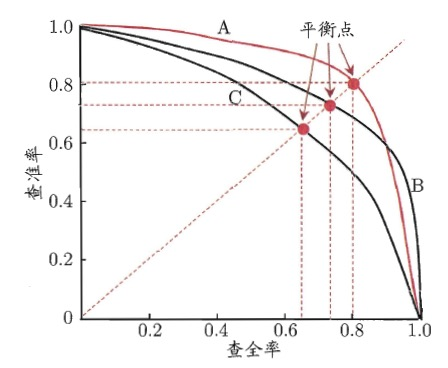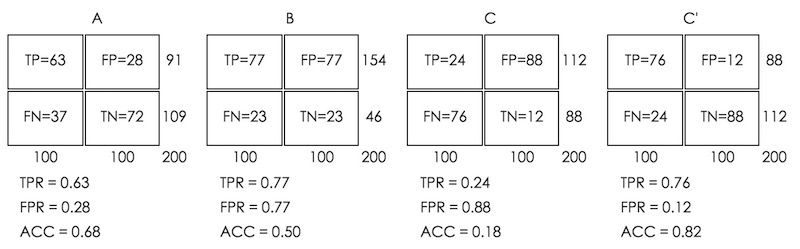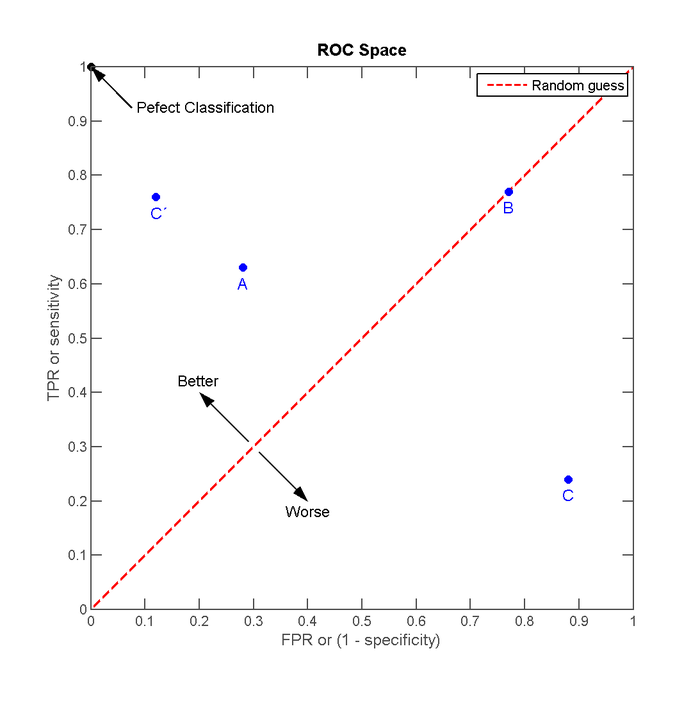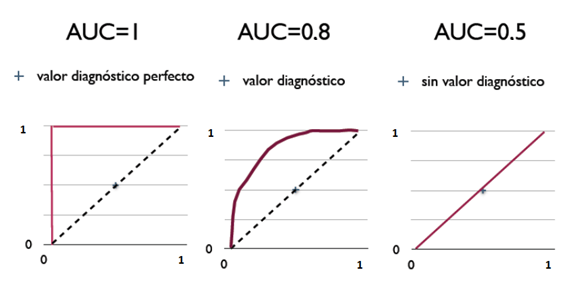# Performance Measures for Machine Learning

## 1 性能度量简介

1、错误率/精度（Accuracy）
2、查准率（准确率，Precision）/查全率（召回率，Recall）
3、ROC曲线/AUC
4、代价曲线（Cost curve）

## 3 查准率、查全率与F1

 真实情况 预测结果 正例 反例 正例 TP (True Positive) FN (False Negative) 反例 FP (False Positive) TN (True Negative)

“False Positive”是“误报”情况，“False Negative”是“漏报”情况。

\begin{aligned} P &= \frac{TP}{TP + FP} \\ R &= \frac{TP}{TP + FN} \end{aligned}

“F1度量（F1 score）”综合地考虑了查准率和查全率，它是基于查准率和查全率的调和平均（harmonic mean）定义的：
$\frac{1}{F1} = \frac{1}{2} \cdot \left(\frac{1}{P} + \frac{1}{R} \right)$

$F1 = \frac{2 \times P \times R}{P + R} = \frac{2 \times TP}{\text{样例总数} + TP - TN}$

### 3.1 PR曲线Figure 1: PR图

## 4 ROC曲线和AUC

ROC 是“受试者工作特征（Receiver Operating Characteristic）”的缩写。ROC曲线首先是由二战中的电子工程师和雷达工程师发明的，用来侦测战场上的敌军载具（飞机、船舰），也就是信号检测理论。

\begin{aligned} TPR &= \frac{TP}{TP + FN} \\ FPR &= \frac{FP}{TN + FP} \end{aligned}Figure 2: 四种预测方法的结果Figure 3: ROC空间的4个例子

### 4.1 ROC曲线下面积（AUC）Figure 4: ROC曲线及AUC值（左边模型最好，中间次之，右边最差）

Created: <2016-09-03 Sat 00:00>

Last updated: <2018-07-19 Thu 13:37>

Creator: Emacs 25.3.1 (Org mode 9.1.4)